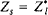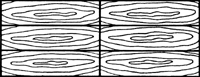# matching

(redirected from Matching problem)
Also found in: Dictionary, Thesaurus, Medical, Financial.
Related to Matching problem: Maximum weight matching

## matching

(impedance matching) Arranging electrical impedances so that maximum power is transferred from one device to another. This occurs when the input impedance of one device equals the output impedance of the other to which it is connected. See also feeder.

## Matching

Used to describe the arrangement of timber veneers, such as book-matching, slip-matching and quarter-matching.

## Matching

in electrical circuit design, the practice that ensures the transmission of electromagnetic energy and signals with the lowest possible reflection factor, signal loss, and distortion. Matching consists in the proper choice of impedances for the source, transmission line, and load. Line and load are ideally matched when the characteristic impedance of the transmission line p is equal to the impedance of the load Zl = Rl + jXl or when Rl = ρ and Xl = 0, where Rl is the active part of the impedance and Xl is the reactive part. Under these circumstances, traveling waves characterized by a standing-wave ratio of unity are established in the transmission line.

For lines with negligibly small electrical losses, matching—and, consequently, the maximum effective transfer of energy from the source to the load—is achieved when the impedance of the source Zs and the load Z, are complex conjugates, that is, whenor Rs = ρ = Rl and Xs = – Xl. In this case, the reactive impedance of the circuit is zero, and the conditions for resonance are satisfied. Such conditions enable electrical systems to operate with better efficiency, for example, with improved use of frequency ranges, better interference rejection, and reduced frequency distortion of radio signals.

Matching is especially important in microwave engineering, where it can be achieved by means of the following: impedance-matching transformers, such as stubs or dielectric sleeves inserted in the transmission line or sections of transmission lines with specially chosen characteristic impedances and lengths; various matching devices that compensate the reactive components of the impedance, such as stubs, phase shifters, and irises, placed in the transmission line; absorbing loads; directional couplers; and various devices for converting from one mode of transmission to another, such as coaxial-waveguide couplers, baluns, and flexible and rotating connections.

The degree of matching can be assessed by measuring the reflection factor and the standing-wave ratio. In practice, matching is considered optimum when the standing-wave ratio in the chosen frequency range does not exceed 1.2 or 1.3 (1.05 for measuring instruments). In some cases, the response of source parameters, such as frequency, power, and noise level, to changes in load, the occurrence of electrical breakdowns in the line, and heating in some sections of the line can serve as indirect indicators of matching.

### REFERENCES

Fizicheskie osnovy elektrotekhniki. Edited by K. M. Polivanov. Moscow-Leningrad, 1950.
Valitov, R. A., and V. N. Sretenskii. Radiotekhnicheskie izmereniia. Moscow, 1970.
Lebedev, I. V. Tekhnika i pribory SVCh, 2nd ed., vol. 1. Moscow, 1970.
Gonorovskii, I. S. Radiotekhnicheskie tsepi i signaly, 2nd ed. Moscow, 1971.

V. N. SRETENSKII

## matching

[′mach·iŋ]
(computer science)
A computer problem-solving method in which the current situation is represented as a schema to be mapped into the desired situation by putting the two in correspondence.
(electricity)
Connecting two circuits or parts together with a coupling device in such a way that the maximum transfer of energy occurs between the two circuits, and the impedance of either circuit will be terminated in its image.
(mathematics)
A set of edges in a graph, no two of which have a vertex in common. Also known as independent edge set.
The bringing of two or more signals or indications into suitable position or condition preliminary to making a measurement, as on a loran indicator or a sky compass.

## matchingmatching, 2
A system of matchboards, or of sheets of wood veneer, arranged to emphasize grain pattern, as in book matching or herringbone matching.
References in periodicals archive ?
We define a new variant of the string matching problem, the [delta][gamma]-order preserving matching problem ([delta][gamma]-OPMP).
A nonregular solution of the nonlinear dynamic disturbance decoupling problem with an application to a complete solution of the nonlinear model matching problem. SIAM J.
They prove that the permutation pattern matching problem is NP-complete when [sigma] has no decreasing subsequence of length 3 and [pi] has no decreasing subsequence of length 4.
Steps 2-6 are the distributed algorithm for maximum weighted matching problem. A link that has been chosen to be in the matching is called matched link.
 Abdulwahab Ali Almazroi, A Fast Hybrid Algorithm Approach for the Exact String Matching Problem Via Berry Ravindran and Alpha Skip Search Algorithms.
Therefore, the proposed traffic scheduling problem can be formulated as a maximum weighted triple matching problem; that is,
Experimental results on the point set matching problem and TSP are presented in Section 3.
Depending on the source, the 'matching problem' may refer to the calculation of any or all of the following:
By matching the students automatically by computer-algorithms PEDALE helps solving the Peer Matching Problem as teachers do not need to match the students manually.
That is why it is crucial to think about potential demand in tackling this kind of matching problem. As with stability, markets cannot be relied upon to deal effectively with this problem on their own.
Comparing with other subgraph matching algorithms, VF2 is superior in solving the subgraph matching problem of graphs .
"If we can solve that matching problem, again, this portfolio which is valued today at \$592 million worth of equity, we think is very, very conservatively priced."

Site: Follow: Share:
Open / Close